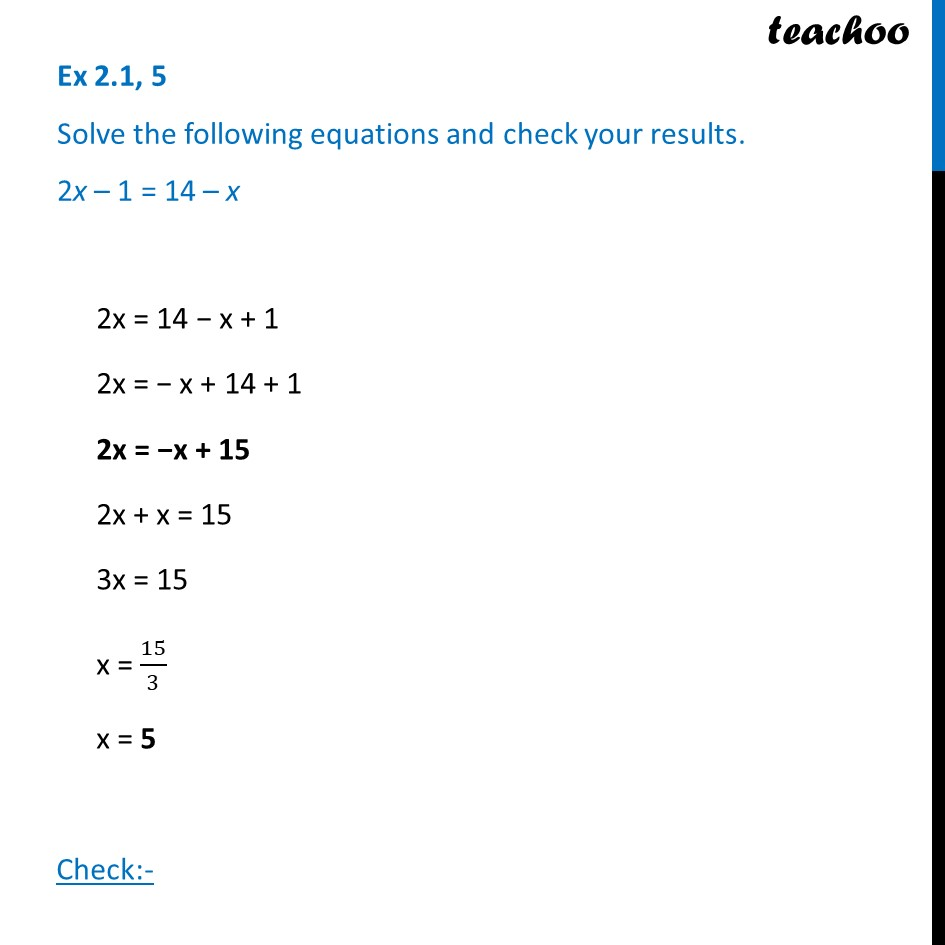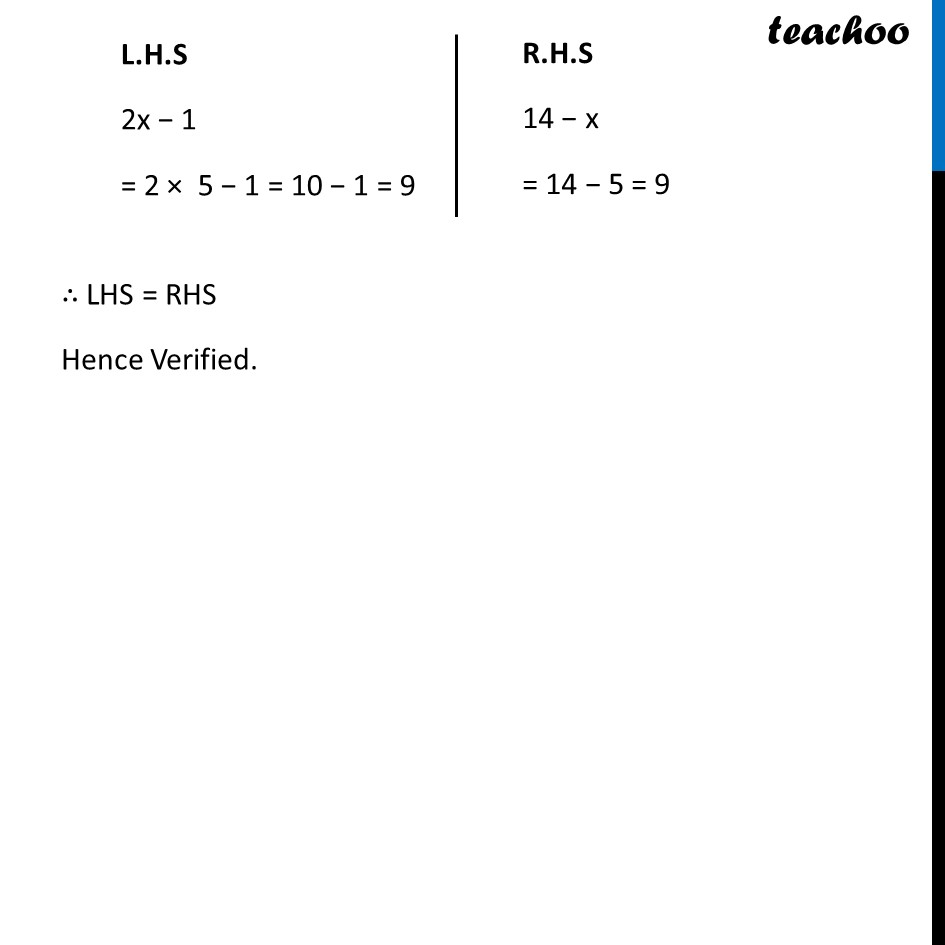Ex 2.1

Chapter 2 Class 8 Linear Equations in One Variable
Serial order wiseLearn in your speed, with individual attention - Teachoo Maths 1-on-1 Class

### Transcript

Ex 2.1, 5 Solve the following equations and check your results. 2x – 1 = 14 – x2x = 14 − x + 1 2x = − x + 14 + 1 2x = −x + 15 2x + x = 15 3x = 15 x = 15/3 x = 5 Check:- L.H.S 2x − 1 = 2 × 5 − 1 = 10 − 1 = 9 R.H.S 14 − x = 14 − 5 = 9 ∴ LHS = RHS Hence Verified.